Publicité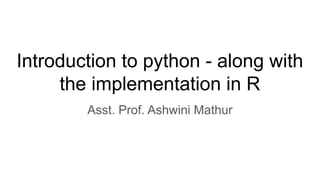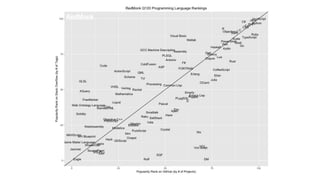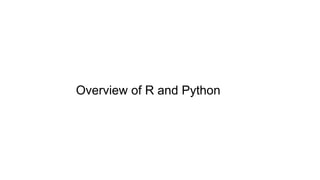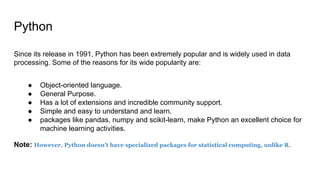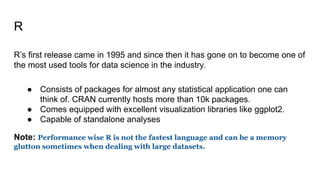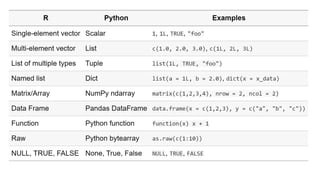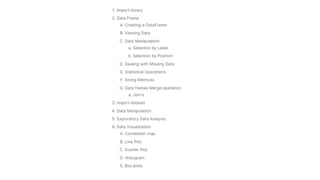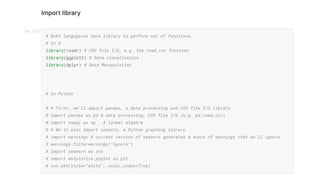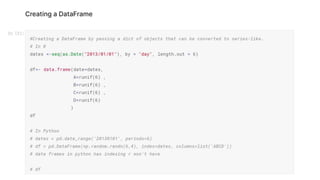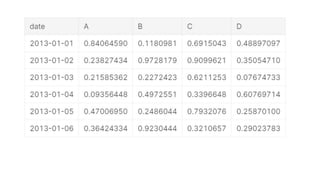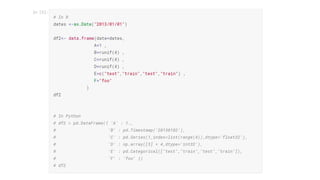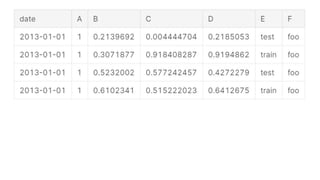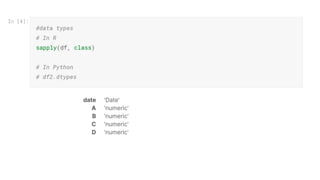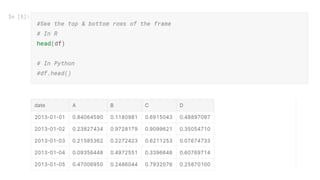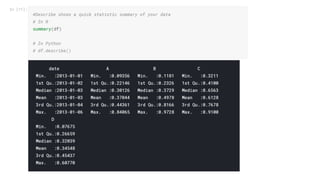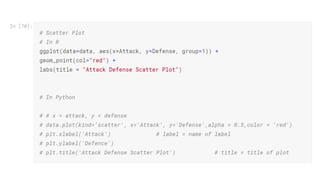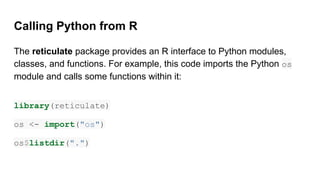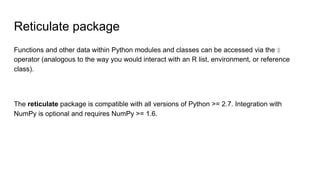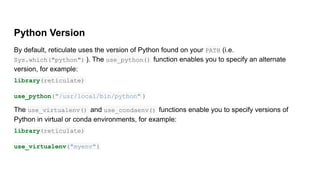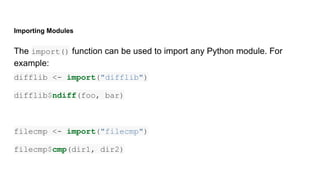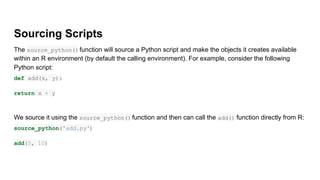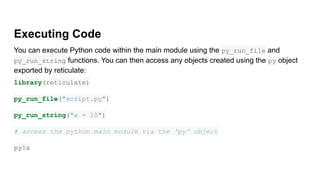1 sur 24
Publicité

### Introduction to python along with the comparitive analysis with r

1. Introduction to python - along with the implementation in R Asst. Prof. Ashwini Mathur
2. Overview of R and Python
3. Python Since its release in 1991, Python has been extremely popular and is widely used in data processing. Some of the reasons for its wide popularity are: ● Object-oriented language. ● General Purpose. ● Has a lot of extensions and incredible community support. ● Simple and easy to understand and learn. ● packages like pandas, numpy and scikit-learn, make Python an excellent choice for machine learning activities. Note: However, Python doesn’t have specialized packages for statistical computing, unlike R.
4. R R’s first release came in 1995 and since then it has gone on to become one of the most used tools for data science in the industry. ● Consists of packages for almost any statistical application one can think of. CRAN currently hosts more than 10k packages. ● Comes equipped with excellent visualization libraries like ggplot2. ● Capable of standalone analyses Note: Performance wise R is not the fastest language and can be a memory glutton sometimes when dealing with large datasets.
5. Calling Python from R The reticulate package provides an R interface to Python modules, classes, and functions. For example, this code imports the Python os module and calls some functions within it: library(reticulate) os <- import("os") os\$listdir(".")
6. Reticulate package Functions and other data within Python modules and classes can be accessed via the \$ operator (analogous to the way you would interact with an R list, environment, or reference class). The reticulate package is compatible with all versions of Python >= 2.7. Integration with NumPy is optional and requires NumPy >= 1.6.
7. Python Version By default, reticulate uses the version of Python found on your PATH (i.e. Sys.which("python") ). The use_python() function enables you to specify an alternate version, for example: library(reticulate) use_python("/usr/local/bin/python" ) The use_virtualenv() and use_condaenv() functions enable you to specify versions of Python in virtual or conda environments, for example: library(reticulate) use_virtualenv("myenv")
8. Importing Modules The import() function can be used to import any Python module. For example: difflib <- import("difflib") difflib\$ndiff(foo, bar) filecmp <- import("filecmp") filecmp\$cmp(dir1, dir2)
9. Sourcing Scripts The source_python()function will source a Python script and make the objects it creates available within an R environment (by default the calling environment). For example, consider the following Python script: def add(x, y): return x + y We source it using the source_python()function and then can call the add() function directly from R: source_python('add.py') add(5, 10)
10. Executing Code You can execute Python code within the main module using the py_run_file and py_run_string functions. You can then access any objects created using the py object exported by reticulate: library(reticulate) py_run_file("script.py") py_run_string("x = 10") # access the python main module via the 'py' object py\$x
11. Demonstration in R Studio : Calling Python Function in R
Publicité